# End-to-End Simulation of HRP UWB IEEE 802.15.4a/z PHY

This example performs end-to-end simulation over an additive white Gaussian noise (AWGN) channel for the high rate pulse repetition frequency (HRP) ultra wideband (UWB) PHY of the IEEE® 802.15.4a/z standard ([ 1 ], [ 2 ]), using the Communications Toolbox™ Library for ZigBee® and UWB add-on.

### Background

The IEEE 802.15.4 standard specifies the PHY and MAC layers of Low-Rate Wireless Personal Area Networks (LR-WPANs) [ 1 ]. The IEEE 802.15.4 PHY and MAC layers are used by other higher-layer standards, such as ZigBee, WirelessHart®, 6LoWPAN, and MiWi.

These PHY schemes are specified in different amendments of the IEEE 802.15.4 standard:

• IEEE 802.15.4a introduced a high rate pulse repetition frequency (HRP) UWB PHY used for ranging and localization [ 1 ].

• IEEE 802.15.4f introduced a low rate pulse repetition frequency (LRP) UWB PHY used for RFID, ranging, and reduced energy consumption [ 1 ].

• IEEE 802.15.4z introduced new enhanced modes for both the HRP and LRP UWB IEEE 802.15.4a/f PHYs [ 2 ].

The HRP UWB PHY has a channel bandwidth of 0.5-1.3 GHz and a pulse duration of 2 ns. The extra short pulse duration makes UWB PHYs suitable for ranging applications, because several ranging techniques rely on calculating the time duration of packet transmission. A finer granularity in the time domain translates to smaller errors in distance estimation.

This example performs end-to-end simulation, computes bit error rate (BER) curves, and demonstrates a tradeoff between reliability and bit rate for these HRP IEEE 802.15.4a/z PHY modes:

1. Higher Pulse Repetition Frequency (HPRF) mode, which was introduced in IEEE 802.15.4z [ 2 ].

2. Base Pulse Repetition Frequency (BPRF) mode, which was introduced in IEEE 802.15.4z [ 2 ] (but can reduce to IEEE 802.15.4a [ 1 ]).

3. IEEE 802.15.4a [ 1 ], which has a lower mean pulse repetition frequency (PRF) and lower data rate than the HPRF and BPRF modes.

This table shows the different modulation schemes, data rates, and number of chips per payload symbol used by these operational modes.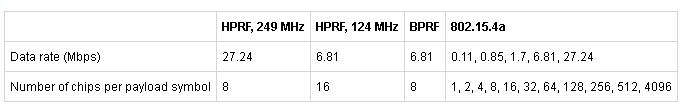### Common Processing Steps

As shown in this PHY chain, the various HRP modes share certain common components.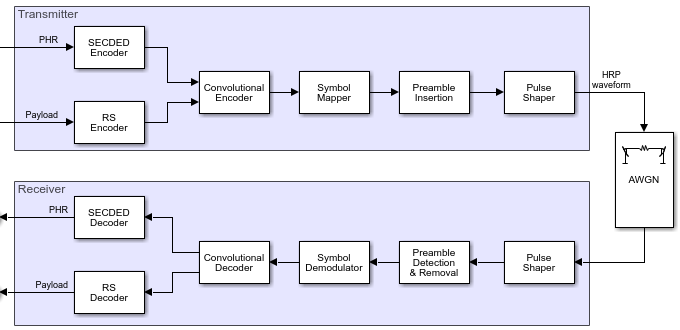The IEEE 802.15.4 and IEEE 802.15.4z standards only specify the transmitter operation ([ 1 ], [ 2 ]). The receiver performs the inverse operations of the transmitter. The receiver implementation does not perform frequency or timing recovery.

SECDED Coding: The PHY header (PHR) is encoded with a single-error-correction, double-error detection (SECDED) Hamming block code. The BER calculation in this example does not use the PHR bits.

RS Coding: The payload is encoded/decoded with a (63, 55) Reed-Solomon code.

Convolutional coding: The payload and the PHR are encoded/decoded with a rate 1/2 convolutional code and a constraint length of 3. An optional rate 1/2 convolutional code with a constraint length 7 is offered for the HPRF mode, but it is not used in this example.

Preamble insertion/removal: A selected code sequence is spread and repeated. The SYNC field consists of this preamble with a start-of-frame delimiter (SFD) appended at the end. The receiver expects the input waveform to begin with the preamble, without any delay that would necessitate preamble detection.

Pulse shaper: The output of symbol mapping and preamble insertion are ternary symbols (-1,0,1). The IEEE 802.15.4a/z standard allows multiple pulse shapes to represent the symbol sequence in the analog domain. Section 15.4 in [ 1 ], [ 2 ] specify RF conformance specifications. In this example, the ternary symbol sequence is passed to a Butterworth filter to create Butterworth pulses. On the receiver side, an integrate-and-dump operation converts the pulses back to ternary symbols.

The main difference between the 3 different modes lies in the Symbol Mapper component (and the respective demodulator). Other differences lie in the PHR format, as well as the length and value of the preamble code sequence and SFD.

### HPRF Mode

In the HPRF mode, the mean pulse repetition frequency (PRF) is either 249.6 MHz or 124.8 MHz, with data rates of 27.24 Mbps or 6.81 Mbps, respectively. In both cases, each symbol duration consists of alternating segments of transmitted chip sequences and guardbands. This code segment generates a plot to show a payload symbol for the 249.6 MHz HPRF mode.

```% Ensure ZigBee/UWB support package is installed: commSupportPackageCheck('ZIGBEE'); msg = randi([0 1], 1000, 1); cfgHPRF = lrwpanHRPConfig(Mode='HPRF',PSDULength=length(msg)); waveHPRF = lrwpanWaveformGenerator(msg,cfgHPRF); fig = lrwpanPlotFrame(waveHPRF,cfgHPRF); hZoomTo1stHPRFPayloadSymbol(fig,cfgHPRF) ```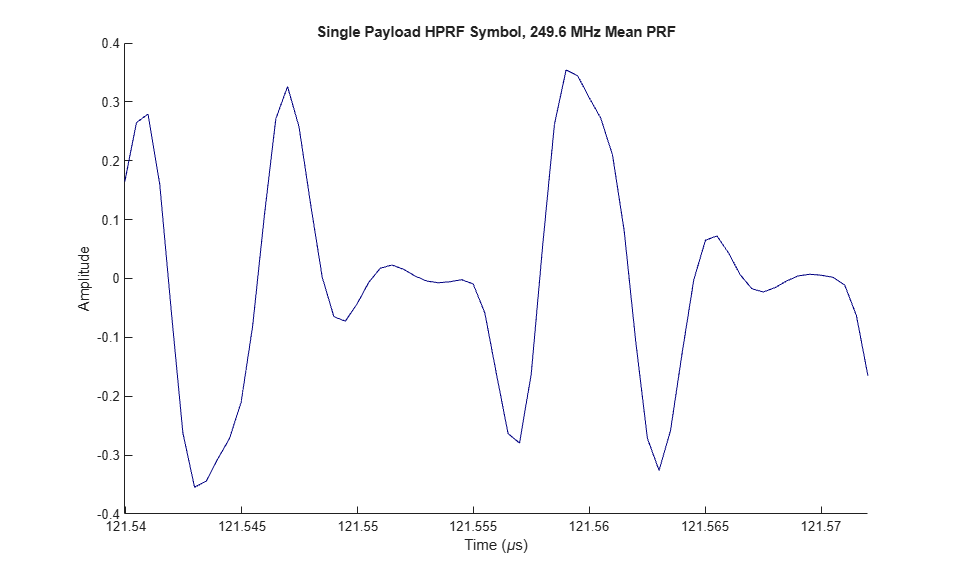Each convolutional codeword is 2 bits long (one parity bit for each systematic). These 2 bits map to 8 payload and 16 PHR bits for the 249.6 MHz mean PRF (see 8 pulses in above plot), and map to 16 payload and 32 PHR bits for the 124.8 MHz mean PRF.

### BPRF Mode

The BPRF mode uses burst-position BPSK modulation (BPM-BPSK). The symbol duration is split into a set of candidate burst positions. Each burst contains a specified number of chips per burst (Ncpb). One candidate burst contains a pattern. All other candidate bursts transmit zeros.

```cfgBPRF = lrwpanHRPConfig(Mode='BPRF',CodeIndex=9); waveBPRF = lrwpanWaveformGenerator(repmat([0; 1],508,1),cfgBPRF); fig = lrwpanPlotFrame(waveBPRF,cfgBPRF); hZoomToBPMBPSKSymbols(fig,cfgBPRF); ```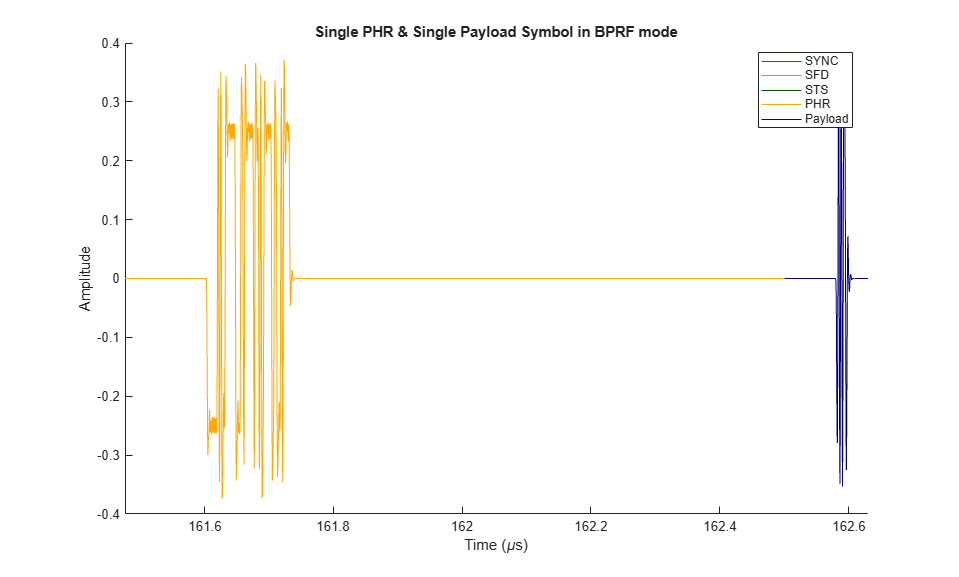In the BPRF mode, the mean PRF is 62.4 MHz and the payload data rate is 6.81 Mbps. The Ncpb for each burst within a symbol is 8 chips, and the number candidate burst positions (Nhop) is 4. The systematic bit reduces the set of candidate positions by 50%, and the active burst is selected among the remaining 2 Nhop based on a spreading (burst-hopping) sequence.

### IEEE 802.15.4a

The IEEE 802.15.4a HRP PHY also uses BPM-BPSK modulation, similar to the BPRF mode. The only difference is that more values are allowed for the mean PRF and data rate combination.

Specifically, mean PRF can be 3.9, 15.6 or 62.4 MHz, while data rate can be 0.11, 0.85, 1.7, 6.81, or 27.24 Mbps. Ncpb can be 1, 2, 4, 8, 16, 32, 64, 128, 256, 512, or 4096. Nhop is 2, 8 or 32. The range of Ncpb values enables transmissions that can be either more aggressive or more conservative than the BPRF mode.

### BER Curve Calculation

For each of the three HRP PHY modes, the example calculates BER over the EcNo range [6,32] in dB (mapped to equivalent SNR values) using end-to-end simulations over an AWGN channel.

For IEEE 802.15.4a, a 15.6 MHz mean PRF is used with a 0.11 Mbps data rate. This combination enables Ncpb equal to 128 and Nhop equal to 8.

```msgLen = 2^10 - 8; msg = randi([0 1],msgLen,1); EcNo = 9:2:35; numSNR = length(EcNo); [berHPRF,berBPRF,ber4a] = deal(zeros(1,numSNR)); % Construct fixed configurations and waveforms for each mode: cfgHPRF = lrwpanHRPConfig(Mode='HPRF',PSDULength=msgLen); waveHPRF = lrwpanWaveformGenerator(msg,cfgHPRF); cfgBPRF = lrwpanHRPConfig(Mode='BPRF',CodeIndex=9,PSDULength=msgLen); waveBPRF = lrwpanWaveformGenerator(msg,cfgBPRF); cfg4a = lrwpanHRPConfig( ... Mode='802.15.4a', ... MeanPRF='15.6MHz', ... DataRate='0.11Mbps', ... CodeIndex=1, ... PSDULength=msgLen); wave4a = lrwpanWaveformGenerator(msg,cfg4a); % Compute BER curve until required number of errors have been found or % maximum number of bits have been simulated. MAXBITS = msgLen*5; MINERRORS = 10; for idx = 1:numSNR fprintf('Calculating BER for EcNo=%d dB\n',EcNo(idx)); errCnt = 0; bitCnt = 0; errHPRF = 0; errBPRF = 0; err4a = 0; while errCnt < MINERRORS && bitCnt < MAXBITS % HPRF mode SNR = EcNo(idx) - 10*log10(cfgHPRF.SamplesPerPulse); noisyHPRF = awgn(waveHPRF,SNR); psduHPRF = lrwpanWaveformDecoder(noisyHPRF,cfgHPRF); errHPRF = biterr(msg, psduHPRF)+errHPRF; % BPRF mode SNR = EcNo(idx) - 10*log10(cfgBPRF.SamplesPerPulse); noisyBPRF = awgn(waveBPRF,SNR); psduBPRF = lrwpanWaveformDecoder(noisyBPRF,cfgBPRF); errBPRF = biterr(msg,psduBPRF)+errBPRF; % Legacy 802.15.4a SNR = EcNo(idx) - 10*log10(cfg4a.SamplesPerPulse); noisy4a = awgn(wave4a,SNR); psdu4a = lrwpanWaveformDecoder(noisy4a,cfg4a); err4a = biterr(msg,psdu4a)+err4a; bitCnt = bitCnt + msgLen; errCnt = min([errHPRF errBPRF err4a]); end berHPRF(idx) = errHPRF/bitCnt; berBPRF(idx) = errBPRF/bitCnt; ber4a(idx) = err4a/bitCnt; end % Plot BER curve figure semilogy(EcNo,berHPRF,'-o',EcNo,berBPRF,'-*',EcNo,ber4a,'-+') legend('HPRF, 27.24 Mbps','BPRF, 6.81 Mbps', ... '15.4a, 0.11 Mbps','Location','southwest') title('BER Curves for UWB IEEE 802.15.4a/z PHY') xlabel('EcNo (dB)') ylabel('BER') grid on ```
```Calculating BER for EcNo=9 dB Calculating BER for EcNo=11 dB Calculating BER for EcNo=13 dB Calculating BER for EcNo=15 dB Calculating BER for EcNo=17 dB Calculating BER for EcNo=19 dB Calculating BER for EcNo=21 dB Calculating BER for EcNo=23 dB Calculating BER for EcNo=25 dB Calculating BER for EcNo=27 dB Calculating BER for EcNo=29 dB Calculating BER for EcNo=31 dB Calculating BER for EcNo=33 dB Calculating BER for EcNo=35 dB ```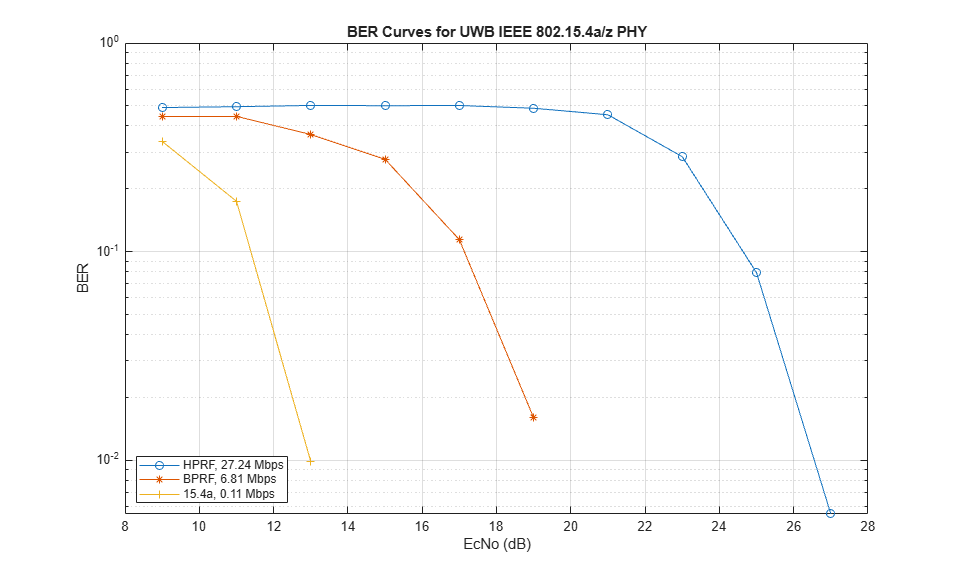The BER curve results demonstrate higher bit error rate for more aggressive modulation schemes and lower BER for more conservative modulation schemes. Lower data rates use more chips for each transmitted convolutional codeword. A higher number of transmitted chips provide more opportunity for error correction, which is similar in concept to using more parity bits in channel coding.

### Further Exploration

The Communications Toolbox Library for ZigBee and UWB add-on contains the following object and functions:

• lrwpanHRPConfig: HRP waveform configuration

• lrwpanWaveformGenerator: Create an IEEE 802.15.4a/z HRP UWB waveform

• lrwpanWaveformDecoder: Decode HRP IEEE 802.15.4a/z UWB waveform

These utilities are undocumented and their API or functionality may change in the future.

### Selected Bibliography

1. "IEEE Standard for Low-Rate Wireless Networks," in IEEE Std 802.15.4-2020 (Revision of IEEE Std 802.15.4-2015), pp.1-800, 23 July 2020, doi: 10.1109/IEEESTD.2020.9144691.

2. "IEEE Standard for Low-Rate Wireless Networks--Amendment 1: Enhanced Ultra Wideband (UWB) Physical Layers (PHYs) and Associated Ranging Techniques," in IEEE Std 802.15.4z-2020 (Amendment to IEEE Std 802.15.4-2020), pp.1-174, 25 Aug. 2020, doi: 10.1109/IEEESTD.2020.9179124.